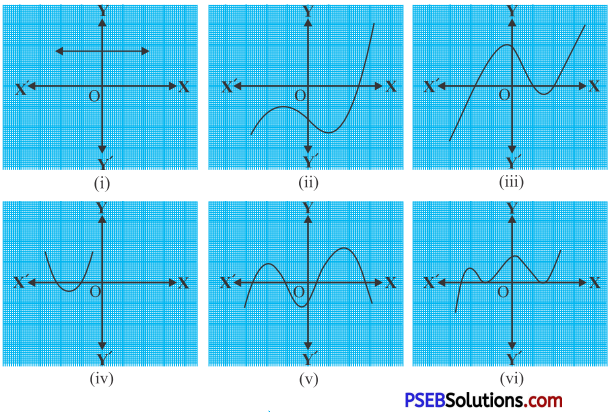# PSEB 10th Class Maths Solutions Chapter 2 Polynomials Ex 2.1

Punjab State Board PSEB 10th Class Maths Book Solutions Chapter 2 Polynomials Ex 2.1 Textbook Exercise Questions and Answers.

## PSEB Solutions for Class 10 Maths Chapter 2 Polynomials Ex 2.1

Question 1.
The graphs of y = p (x) are given in Fig. below, for some polynomials p(x). Find the number of zeroes of p (x), in each case.Solution:
The graphs of y = p (x) are given in figure above, for some polynomials p (x). The number of zeroes of p (x) in each case are given below:
(i) From the graph, it is clear that it does not meet x-axis at any point.
Therefore, it has NIL; no. of zeroes.

(ii) From the graph, it is clear that it meets x- axis at only one point.
Therefore, it has only one no. of zeroes.

(iii) From the graph, it is clear that it meets x-axis at three points.
Therefore, it has three no. of zeroes.

(iv) From the graph, it is clear that it meets x- axis at two points.
Therefore, it has two no. of zeroes.

(v) From the graph, it is clear that it meets x- axis at four points.
Therefore, it has four no. of zeroes.

(vi) From the graph, it is clear that it meets pr-axis at three points.
Therefore, it has three no. of zeroes.